Electron. J. Diff. Eqns., Vol. 2006(2006), No. 111, pp. 1-9.

### On the first eigenvalue of the Steklov eigenvalue problem for the infinity Laplacian An Le

Abstract:
Let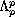be the best Sobolev embedding constant of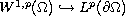, where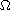is a smooth bounded domain in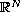. We prove that as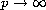the sequence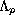converges to a constant independent of the shape and the volume of, namely 1. Moreover, for any sequence of eigenfunctions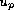(associated with), normalized by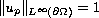, there is a subsequence converging to a limit function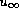which satisfies, in the viscosity sense, an-Laplacian equation with a boundary condition.

Submitted August 4, 2006. Published September 18, 2006.
Math Subject Classifications: 35J50, 35J55, 35J60, 35J65, 35P30.
Key Words: Nonlinear elliptic equations; eigenvalue problems; p-Laplacian; nonlinear boundary condition; Steklov problem; viscosity solutions.

Show me the PDF file (220K), TEX file, and other files for this article.An Lê Department of Mathematics and Statistics Utah State University Logan, Utah 84322, USA email: anle@cc.usu.edu